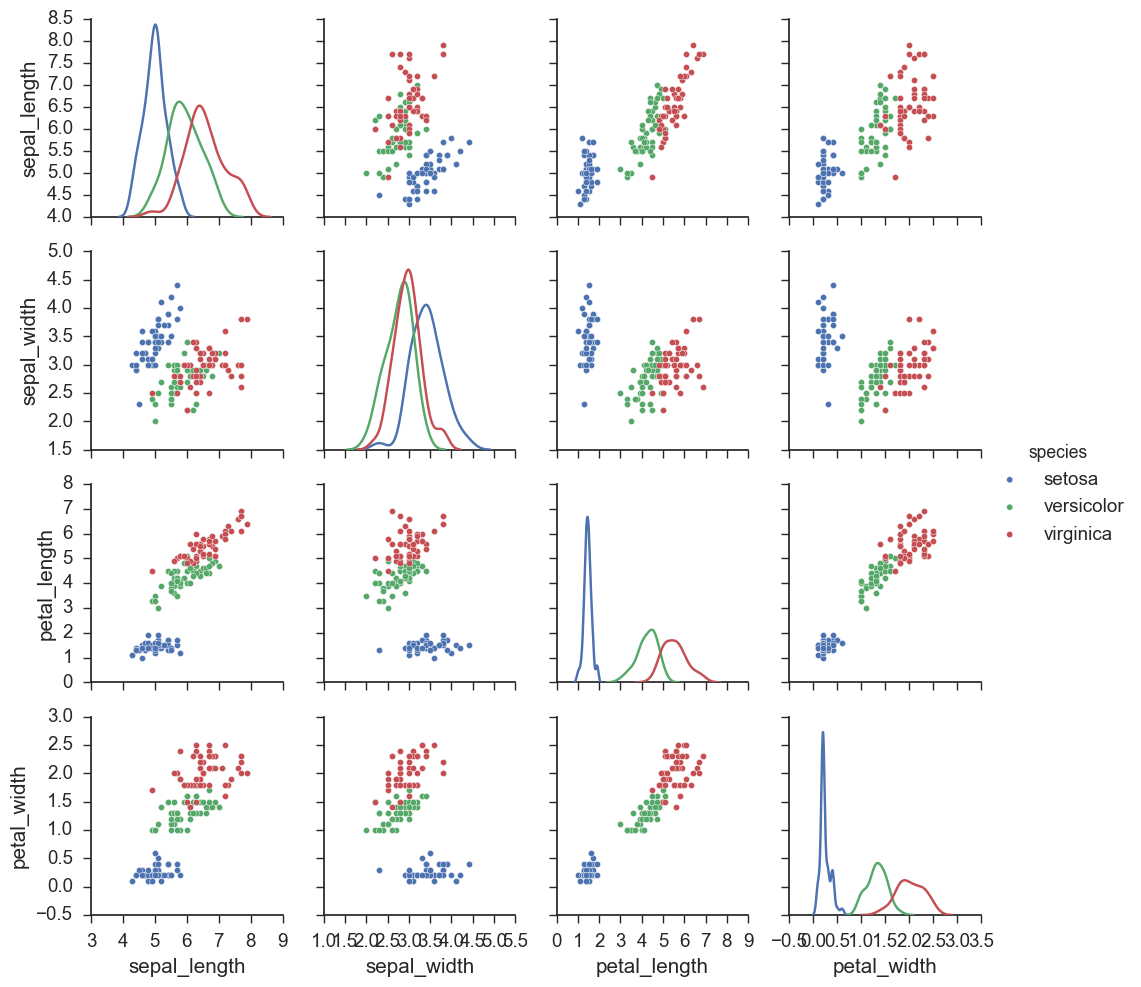# Pair plots

A pairs plot allows us to see both distribution of single variables and relationships between two variables.

Simple 2D Scatter plot is used to understand the relationship or pattern between two variables or dimensions in our dataset.

A 3D plot will be used for three variables or dimensions.

However, what would do if we have more than 3 dimensions or features in our dataset as we humans do have the capability to visualize more than 3 dimensions?

One solution to this problem is pair plots. It is one of the most effective starting tools. They are used to plot features when we have more than three dimensions. As the name suggests we actually do pairs of features and plot them all.

For example, let’s say we have four features ‘sepal_length’, ‘sepal_width’, ‘petal_length’ and ‘petal_width’ in our iris dataset. In that case, we will have 4C2 plots i.e. 6 unique plots. The pairs in this case will be :

1. (sepal_length, sepal_width)
2. (sepal_length, petal_length)
3. (sepal_length, petal_width)
4. (sepal_width, petal_length)
5. (sepal_width, petal_width) and
6. (petal_length, petal_width).

So, here instead of trying to visualize four dimensions which is not possible. We will look into 6 2D plots and try to understand the 4-dimensional data in the form of a matrix.

We can just write one line of code and we have our pair plots.

```import matplotlib.pyplot as plt
import seaborn as sns

sns.set_style("whitegrid");
sns.pairplot(iris, hue = "species", size = 3);
plt.show()
# NOTE: the diagonal elements are PDFs for each feature.```

Output :### As Seen Above, The Pair Plots Can Be Divided Into Three Parts:

• The diagonal plot which showcases the histogram. The histogram allows us to see the PDF/Probability distribution of a single variable
• Upper triangle and lower triangle which shows us the scatter plot.
• The scatter plots show us the relationship between the features. These upper and lower triangles are the mirror image of each other.

Change the bar plot to lines graphs :

`plot = sns.pairplot(iris, hue='species', diag_kind = 'kde')`

The density plots on the diagonal make it easier to compare distributions between the continents than stacked bars.

Output :Change the palette of the plot :

`plot = sns.pairplot(iris, hue='species', diag_kind='kde',palette='husl')`

Output :Pairs plots are a powerful tool to quickly explore distributions and relationships in a dataset.

Notes :

Pair plot will only plot the variables which are numerical. The variables which are of String type, by default pair plot won’t plot automatically.

If i want plot that non -integer variable in graph then I have to explicitly mention in parameter Vars .

You can’t plot the non-numerical variables. If you want to plot, then you need to encode it as numerical. However, Seaborn will encode internally and assign a label to each unique value in the non-numerical values.

`snb.pairplot(iris, vars = [“petal_length”,”species”]).`

** Pair plot already adds a legend outside the plot matrix and the default location is vertically oriented on the right outside as seen in the plot.

https://seaborn.pydata.org/generated/seaborn.pairplot.html

How to change colors in legend? means if we don’t want default colors ??

Using Palette parameter in seaborn , we can change the default colors of legends:
for example:

```pal = [ ‘red’, ‘green’, ‘blue’]
sns.pairplot(iris, hue = ”species”, palette = pal,size=3)```

Clarity of “hue” property :

Whenever we want to draw a pair-plot, we need the dataframe on whose numerical columns we have to apply pair-plot. But for clear understanding and interpretation, we use different colors for each category data points. In order to select that particular column on the basis of whose values we have to apply colors, we specify that column name “hue” property. In this example, as our intention is to specify different colors for different species, we are using “species” column in “hue”.

Pairplot plots only numerical columns and in ‘hue’ parameter we can provide the categorical column.

Pairplot is usually a grid of plots for each variable in your dataset. Hence you can quickly see how all the variables are related. This can help to infer which variables are useful, which have skewed distribution etc.

Cons :

If you have d features, you will have a pair plot of size dxd cells where each cell is a plot between a pair of features. So, if you have a high dimensional (say d=1000) dataset, just looking at the plot would become very hard as you will have to go through 1000*1000 plots which is humanly impossible. So, pair plots are hard to use when we have high dimensional data. Wee will learn techniques like PCA, t-SNE later in the course to visualize high dimensional data.

References :

https://etav.github.io/python/pairs_plot_python_seaborn.html

http://zerosnones.net/pair-plots/

https://etav.github.io/#Python